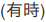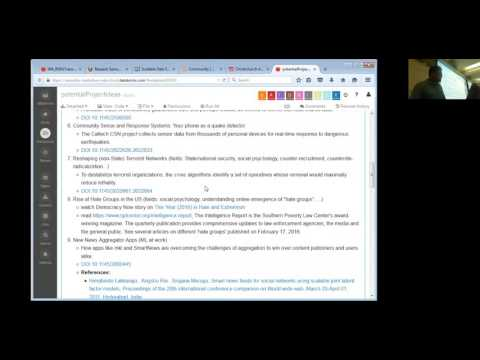// Databricks notebook source exported at Sat, 18 Jun 2016 04:23:16 UTC

# Scalable Data Science

### prepared by Raazesh Sainudiin and Sivanand Sivaram

The html source url of this databricks notebook and its recorded Uji:# HOMEWORK notebook - RDDs Transformations and Actions

Just go through the notebook and familiarize yourself with these transformations and actions.

##### 1. Perform the `takeOrdered` action on the RDD

To illustrate `take` and `takeOrdered` actions, let’s create a bigger RDD named `rdd0_1000000` that is made up of a million integers from 0 to 1000000.
We will `sc.parallelize` the `Seq` Scala collection by using its `.range(startInteger,stopInteger)` method.

``````
val rdd0_1000000 = sc.parallelize(Seq.range(0, 1000000)) // <Shift+Enter> to create an RDD of million integers: 0,1,2,...,10^6

``````
``````
rdd0_1000000.take(5) // <Ctrl+Enter> gives the first 5 elements of the RDD, (0, 1, 2, 3, 4)

``````

`takeordered(n)` returns `n` elements ordered in ascending order (by default) or as specified by the optional key function, as shown below.

``````
rdd0_1000000.takeOrdered(5) // <Shift+Enter> is same as rdd0_1000000.take(5)

``````
``````
rdd0_1000000.takeOrdered(5)(Ordering[Int].reverse) // <Ctrl+Enter> to get the last 5 elements of the RDD 999999, 999998, ..., 999995

``````
``````
// HOMEWORK: edit the numbers below to get the last 20 elements of an RDD made of a sequence of integers from 669966 to 969696
sc.parallelize(Seq.range(0, 10)).takeOrdered(5)(Ordering[Int].reverse) // <Ctrl+Enter> evaluate this cell after editing it for the right answer

``````
##### 2. More examples of `map`
``````
val rdd = sc.parallelize(Seq(1, 2, 3, 4))    // <Shift+Enter> to evaluate this cell (using default number of partitions)

``````
``````
rdd.map( x => x*2) // <Ctrl+Enter> to transform rdd by map that doubles each element

``````

To see what’s in the transformed RDD, let’s perform the actions of `count` and `collect` on the `rdd.map( x => x*2)`, the transformation of `rdd` by the `map` given by the closure `x => x*2`.

``````
rdd.map( x => x*2).count()    // <Shift+Enter> to perform count (action) the element of the RDD = 4

``````
``````
rdd.map( x => x*2).collect()    // <Shift+Enter> to perform collect (action) to show 2, 4, 6, 8

``````
``````
// HOMEWORK: modify the '???' in the code below to collect and display the square (x*x) of each element of the RDD
// the answer should be Array[Int] = Array(1, 4, 9, 16) Press <Cntrl+Enter> to evaluate the cell after modifying '???'
sc.parallelize(Seq(1, 2, 3, 4)).map( x => ???).collect()

``````
##### 3. More examples of `filter`

Let’s declare another `val` RDD named `rddFiltered` by transforming our first RDD named `rdd` via the `filter` transformation `x%2==0` (of being even).

This filter transformation based on the closure `x => x%2==0` will return `true` if the element, modulo two, equals zero. The closure is automatically passed on to the workers for evaluation (when an action is called later). So this will take our RDD of (1,2,3,4) and return RDD of (2, 4).

``````
val rddFiltered = rdd.filter( x => x%2==0 )    // <Ctrl+Enter> to declare rddFiltered from transforming rdd

``````
``````
rddFiltered.collect()    // <Ctrl+Enter> to collect (action) elements of rddFiltered; should be (2, 4)

``````
##### 4. More examples of `reduce`
``````
val rdd = sc.parallelize(Array(1,2,3,4,5))

``````
``````
rdd.reduce( (x,y)=>x+y ) // <Shift+Enter> to do reduce (action) to sum and return Int = 15

``````
``````
rdd.reduce( _ + _ )    // <Shift+Enter> to do same sum as above and return Int = 15 (undescore syntax)

``````
``````
rdd.reduce( (x,y)=>x*y ) // <Shift+Enter> to do reduce (action) to multiply and return Int = 120

``````
``````
val rdd0_1000000 = sc.parallelize(Seq.range(0, 1000000)) // <Shift+Enter> to create an RDD of million integers: 0,1,2,...,10^6

``````
``````
rdd0_1000000.reduce( (x,y)=>x+y ) // <Ctrl+Enter> to do reduce (action) to sum and return Int 1783293664

``````
``````
// the following correctly returns Int = 0 although for wrong reason
// we have flowed out of Int's numeric limits!!! (but got lucky with 0*x=0 for any Int x)
// <Shift+Enter> to do reduce (action) to multiply and return Int = 0
rdd0_1000000.reduce( (x,y)=>x*y )

``````
``````
// <Ctrl+Enter> to do reduce (action) to multiply 1*2*...*9*10 and return correct answer Int = 3628800
sc.parallelize(Seq.range(1, 11)).reduce( (x,y)=>x*y )

``````

CAUTION: Know the limits of your numeric types!

The minimum and maximum value of `Int` and `Long` types are as follows:

``````
(Int.MinValue , Int.MaxValue)

``````
``````
(Long.MinValue, Long.MaxValue)

``````
``````
// <Ctrl+Enter> to do reduce (action) to multiply 1*2*...*20 and return wrong answer as Int = -2102132736
//  we have overflowed out of Int's in a circle back to negative Ints!!! (rigorous distributed numerics, anyone?)
sc.parallelize(Seq.range(1, 21)).reduce( (x,y)=>x*y )

``````
``````
//<Ctrl+Enter> we can accomplish the multiplication using Long Integer types
// by adding 'L' ro integer values, Scala infers that it is type Long
sc.parallelize(Seq.range(1L, 21L)).reduce( (x,y)=>x*y )

``````

As the following products over Long Integers indicate, they are limited too!

``````
// <Shift+Enter> for wrong answer Long = -8718968878589280256 (due to Long's numeric limits)
sc.parallelize(Seq.range(1L, 61L)).reduce( (x,y)=>x*y )

``````
``````
// <Cntrl+Enter> for wrong answer Long = 0 (due to Long's numeric limits)
sc.parallelize(Seq.range(1L, 100L)).reduce( (x,y)=>x*y )

``````

##### 5. Let us do a bunch of transformations to our RDD and perform an action
• start from a Scala `Seq`,
• `sc.parallelize` the list to create an RDD,
• `filter` that RDD, creating a new filtered RDD,
• do a `map` transformation that maps that RDD to a new mapped RDD,
• and finally, perform a `reduce` action to sum the elements in the RDD.

This last `reduce` action causes the `parallelize`, the `filter`, and the `map` transformations to actually be executed, and return a result back to the driver machine.

``````
sc.parallelize(Seq(1, 2, 3, 4))    // <Ctrl+Enter> will return Array(4, 8)
.filter(x => x%2==0)             // (2, 4) is the filtered RDD
.map(x => x*2)                   // (4, 8) is the mapped RDD
.reduce(_+_)                     // 4+8=12 is the final result from reduce

``````
##### 6. Transform the RDD by `distinct` to make another RDD

Let’s declare another RDD named `rdd2` that has some repeated elements to apply the `distinct` transformation to it. That would give us a new RDD that only contains the distinct elements of the input RDD.

``````
val rdd2 = sc.parallelize(Seq(4, 1, 3, 2, 2, 2, 3, 4))    // <Ctrl+Enter> to declare rdd2

``````

Let’s apply the `distinct` transformation to `rdd2` and have it return a new RDD named `rdd2Distinct` that contains the distinct elements of the source RDD `rdd2`.

``````
val rdd2Distinct = rdd2.distinct() // <Ctrl+Enter> transformation: distinct gives distinct elements of rdd2

``````
``````
rdd2Distinct.collect()    // <Ctrl+Enter> to collect (action) as Array(4, 2, 1, 3)

``````
##### 7. more flatMap
``````
val rdd = sc. parallelize(Array(1,2,3)) // <Shift+Enter> to create an RDD of three Int elements 1,2,3

``````

Let us pass the `rdd` above to a map with a closure that will take in each element `x` and return `Array(x, x+5)`. So each element of the mapped RDD named `rddOfArrays` is an `Array[Int]`, an array of integers.

``````
// <Shift+Enter> to make RDD of Arrays, i.e., RDD[Array[int]]
val rddOfArrays = rdd.map( x => Array(x, x+5) )

``````
``````
rddOfArrays.collect() // <Ctrl+Enter> to see it is RDD[Array[int]] = (Array(1, 6), Array(2, 7), Array(3, 8))

``````

Now let’s observer what happens when we use `flatMap` to transform the same `rdd` and create another RDD called `rddfM`.

Interestingly, `flatMap` flattens our `rdd` by taking each `Array` (or sequence in general) and truning it into individual elements.

Thus, we end up with the RDD `rddfM` consisting of the elements (1, 6, 2, 7, 3, 8) as shown from the output of `rddfM.collect` below.

``````
val rddfM = rdd.flatMap(x => Array(x, x+5))    // <Shift+Enter> to flatMap the rdd using closure (x => Array(x, x+5))

``````
``````
rddfM.collect    // <Ctrl+Enter> to collect rddfM = (1, 6, 2, 7, 3, 8)

``````

Updated: